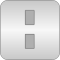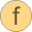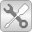# Vectors

Library of functions operating on vectors# Package ContentstoString Convert a real vector in to a string representationisEqual Determine if two Real vectors are numerically identicalnorm Return the p-norm of a vectorlength Return length of a vector (better as norm(), if further symbolic processing is performed)normalize Return normalized vector such that length = 1 and prevent zero-division for zero vectornormalizeWithAssert Return normalized vector such that length = 1 (trigger an assert for zero vector)reverse Reverse vector elements (e.g., v becomes last element)sort Sort elements of vector in ascending or descending orderfind Find element in a vectorinterpolate Interpolate linearly in a vectorrelNodePositions Return vector of relative node positions (0..1)Utilities Utility functions that should not be directly utilized by the user

# Information

This information is part of the Modelica Standard Library maintained by the Modelica Association.

#### Library content

This library provides functions operating on vectors:

• toString(v) - returns the string representation of vector v.
• isEqual(v1, v2) - returns true if vectors v1 and v2 have the same size and the same elements.
• norm(v,p) - returns the p-norm of vector v.
• length(v) - returns the length of vector v (= norm(v,2), but inlined and therefore usable in symbolic manipulations)
• normalize(v) - returns vector in direction of v with length = 1 and prevents zero-division for zero vector.
• reverse(v) - reverses the vector elements of v.
• sort(v) - sorts the elements of vector v in ascending or descending order.
• find(e, v) - returns the index of the first occurrence of scalar e in vector v.
• interpolate(x, y, xi) - returns the interpolated value in (x,y) that corresponds to xi.
• relNodePositions(nNodes) - returns a vector of relative node positions (0..1).